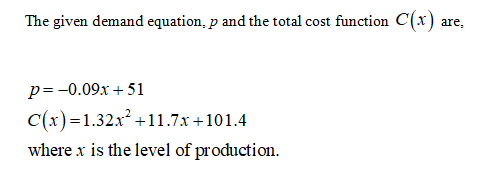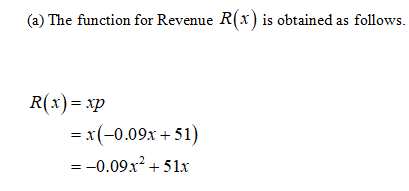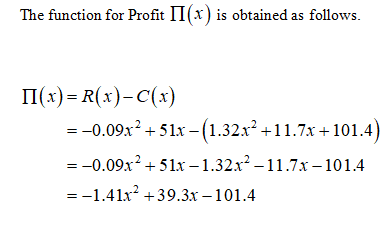# If the demand curve for a particular commodity is p = −0.09x + 51 and thetotal cost function C(x) = 1.32x2 + 11.7x + 101.4,where x is the level of production. Find(a) The revenue R(x) and profit Π(x).(b) All values of x for which production of the commodity is profitabl

Question
6 views

If the demand curve for a particular commodity is p = −0.09x + 51 and the
total cost function C(x) = 1.32x
2 + 11.7x + 101.4,where x is the level of production. Find
(a) The revenue R(x) and profit Π(x).
(b) All values of x for which production of the commodity is profitabl

check_circle

Step 1Step 2Step 3...

### Want to see the full answer?

See Solution

#### Want to see this answer and more?

Solutions are written by subject experts who are available 24/7. Questions are typically answered within 1 hour.*

See Solution
*Response times may vary by subject and question.
Tagged in